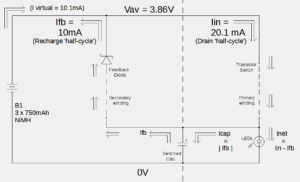# How to Achieve a 200% Conversion of Energy into Work – The Ringwood Energy Recycler Experiment

The following post has been submitted by Sandy Robson

DISCLAIMER

– this is not an attempt to create, or work towards a perpetuum mobile; the circuit used in these tests includes all the usual losses, it is the configuration and interaction of the whole system (battery + circuit) which exhibits interesting behaviour

– trying to quantify values for power levels and energy conversion can be tricky when current is switched through coils and LEDs; so, to avoid contention, the results described here have been obtained (as far as possible) by quantifying and comparing DC values, quantities of charge, and durations of time

INTRODUCTION

The recharging of secondary batteries (or cells) requires electrical energy to be supplied, converted as work done in driving current through the cells; as a result of this work done, chemical energy becomes stored within the cells, available to be be converted back into electrical energy when required

A fully-charged 3 cell 750mAh NiMH battery, for example, could contain up to approximately 10000 Joules of energy

You might reasonably expect that when such a battery is then used to supply electrical power that we would only be able to convert a maximum of 10000 Joules of energy as work done

It appears, however, that it is possible to effectively double the total amount of work converted from the energy supplied

For example, we can arrange for part of the energy provided by the battery to be converted, say, to light, by means of a simple switched-mode power supply type circuit, driving a cluster of a few LEDs; some energy will be converted to heat as losses in the system, but any remaining part of the energy can be collected and used to do work in recharging a battery

The circuit used in these tests is not particularly unusual – it’s a simple blocking oscillator and it has a work-efficiency of approximately 85% (quantifiable-work performed for total energy supplied)

Unquantified losses include resistive heat-loss in the switching transistor & diode, coil windings, and transistor biasing

BENCHMARKING

The battery capacity can be characterised by discharging it using a resistor with a known value

As an example, using a battery with 3 NiMH cells of 750mAh rated capacity, a fully charged battery supplied a 268 ohm (measured) resistor with an average power of 14.4mA x 3.86V for 42.5 hours, converting a total of 8504 Joules of energy

CONVENTIONAL SETUP

When the discharge resistor is replaced with the preliminary circuit, the quantifiable work consists of two parts:-
– converting 3105 Joules to illuminate some LEDs
– recharging a spare battery (in this case, not the supply battery) by using the 4238 Joules of energy which is being temporarily stored in the circuit as a by-product of the oscillator operation

The circuit provides this quantifiable work for a total of 30.5 hours, drawing an average of 20.1mA at 3.86V to draw a total of 8603 Joules (approximately matching the total energy, 8504 Joules, converted by the nominal 270 ohm resistor)

In this mode, the circuit draws the total energy available and converts this to 7343 Joules of quantifiable work, giving a quantifiable-work efficiency of 85%

so far, so expected

SETUP WITH ‘RECYCLED’ ENERGY

When the circuit is connected in ‘feedback to supply’ mode, however (see Fig. 1 in the accompanying Data PDF file for an overview of the circuit current paths), we see some interesting behaviour emerging: the most obvious is that the circuit will continue to operate for almost twice the duration of the conventional, non-feedback mode – the circuit now operates for a total of 60.5 hours, whilst drawing the same real average power, 20.1mA at 3.86V, (compared to 30.5 hours duration for the non-feedback mode) with the loads remaining the same values.

[Note that the circuit can operate at the same power drain for longer because it is constantly re-charging its supply with a proportion of the energy which has been input – the actual supply current drained is pulsed, with an average of 20.1mA, whilst 10mA av. is switched back into the battery, being interleaved each cycle similar to time-division-multiplexed operation (see Fig. 2 in the Data PDF file), resulting in a ‘virtual’ current drain from the battery of (20.1mA – 10.1mA) = 10mA av.]

The total energy drawn by the circuit is now 16898 Joules

The efficiency of the oscillator circuit compared to its total energy drawn hasn’t changed essentially:
the quantifiable work has increased to 14566 Joules, and these values give an efficiency of 14566 / 16898 = 86%

The overall system efficiency for quantifiable work from the original energy in the battery, however, is now 14566 / 8504 = 170%

The total system efficiency for conversion of energy in the whole system (battery + circuit) now becomes: 16898 / 8504 = 199%

CONCLUSIONS

The system is recycling its input energy by a factor of 1.99

The ‘feedback to supply’ mode has extended the duration for circuit operation and enabled the amount of useful energy available for the LEDs to be approximately doubled compared to the ‘no feedback’ arrangement.

Although the total work converted by the switching circuit LEDs, on their own, remains less than the original supply of energy, and the work-efficiency of those circuit LEDs matches that of a passive resistor-driven LED arrangement, the ‘With-Feedback’ current arrangement still enables the circuit to produce a level of light output slightly greater than the passive DC-drive arrangement but for approximately 34% longer duration (45 hours duration for the passive drive, 60.5 hours for the ‘With-Feedback’ circuit).

This resulting system behaviour provides a worthwhile gain, which has been enabled by the 200% conversion to work of the original store of energy in the battery.

s robson
27 May 2019

ringwood_recycler_results_190527